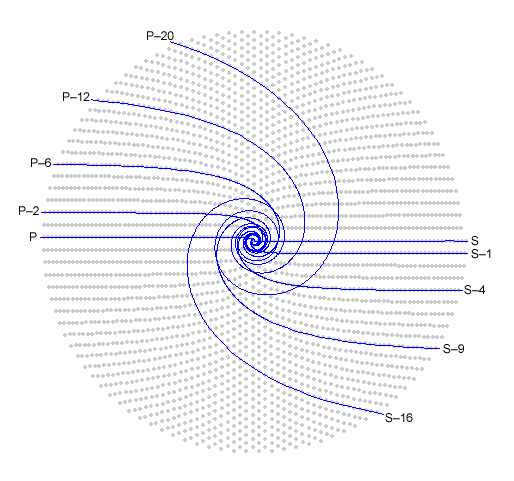NumberSpiral.com
 Home Download Contact Links Next page

CONTENTS2.   Product Curves

 On the previous page we saw that primes tend to line up in curves on the number spiral. In fact, the whole spiral is made of curves of this kind, and every integer belongs to an infinite number of them. The simplest curve of this type (the one with least curvature) is the line of perfect squares, marked here in blue:Figure 1

 For reasons that will become clear as we go on, we need to think of the numbers on this line in terms of their factors. 1 is really 1 x 1; 4 is really 2 x 2; 9 is really 3 x 3; etc. For convenience, I'll call this line "curve S" for '"squares." Here's another example:

DetailsFig. 1 shows the values of  n2 for non-negative integer n. The blue curves should not be taken as graphs of continuous functions. They only indicate sequences of integers. There are no numbers in the white gaps between the gray coils of the spiral.VortexTo draw this curve with Vortex, enter "P" in the command window.

DetailsFig. 2 shows  n2 + n.Figure 2

 The factors of numbers on this second curve are 1 x 2, 2 x 3, 3 x 4, etc. The difference between factors is always 1. Such numbers are called pronics, so I'll name this second line "Curve P".

 In curve S, the difference between factors is zero. In curve P, it is one. Is there also a curve where the difference is two? Here it is:Figure 3

VortexTo draw this curve with Vortex, enter "S–1" in the command window.

DetailsFig. 3 shows  n2 – 1.Factors of numbers on the third curve are 1 x 3, 2 x 4, 3 x 5, etc. These numbers are one less than those on curve S, so this curve is called S–1. One more example:Figure 4

VortexTo draw this curve with Vortex, enter "P–2" in the command window.

DetailsFig. 4 shows  n2 + n – 2.Factors of numbers on the fourth curve are 1 x 4, 2 x 5, 3 x 6, etc. The factors have a difference of 3. For obvious reasons, this curve is called P–2. Note that 4 has appeared on two curves. But it meant two different things. The first time it meant "2 x 2". The second time it meant "1 x 4."

 We can continue this way forever, increasing the difference between factors and finding new curves. Here are the first ten such curves:Figure 5

 I call these sequences product curves because they consist of products of factors with a fixed difference between them. Every integer lies on at least one of these curves — the curve that has zero as its first member and the integer itself as the second. Composites lie on more than one of these curves. A prime is an integer that lies on only one product curve. It's always the second member of that curve (following zero).DetailsCurve S is n2, so the product curves on the right side of the spiral are n2 – 1, n2 – 4, n2 – 9, etc. Curve P is n2 + n so the curves on the left side are n2 + n – 2, n2 + n – 6, n2 + n – 12, etc. These curves have many interesting properties.. I'll point out just a few. As the S-minus curves extend outward, the angles subtended by the numbers on them approach zero rotations (zero degrees). Therefore the fractional parts of the square roots of integers on those curves must approach zero. Similarly, the angles subtended by the numbers on Curve P and all the P-minus curves approach an angle of 1/2 rotation (180 degrees). Therefore the fractional parts of the square roots of integers on those curves must approach 1/2. The differences between S and the "S-minus curves" are perfect squares. Similarly, the differences between the pronics and the P-minus curves are pronics. This is another way of saying that these numbers can be factored in the ways we have already described. Odd curves (those with factors that differ by odd numbers) are offset from curve P, and even curves are offset from curve S. The nth product curve on the even (square) side, counting curve S as zero, has a difference between factors of 2n and an offset from curve S of n2. The nth product curve on the odd side has difference 2n + 1 and offset n2 + n. I said a moment ago that the blue lines do not represent continuous functions, but obviously I'm using a continuous function to draw them, and of course that function is related to the integer sequences.. I'll explain the formula in a later section but for now, I'll just point out that the number of revolutions made by a blue curve before it straightens out is equal to half the difference between factors.1  2  3  4  5  6  7  8  9  10   NextCopyright © 2003, 2007 Robert Sacks